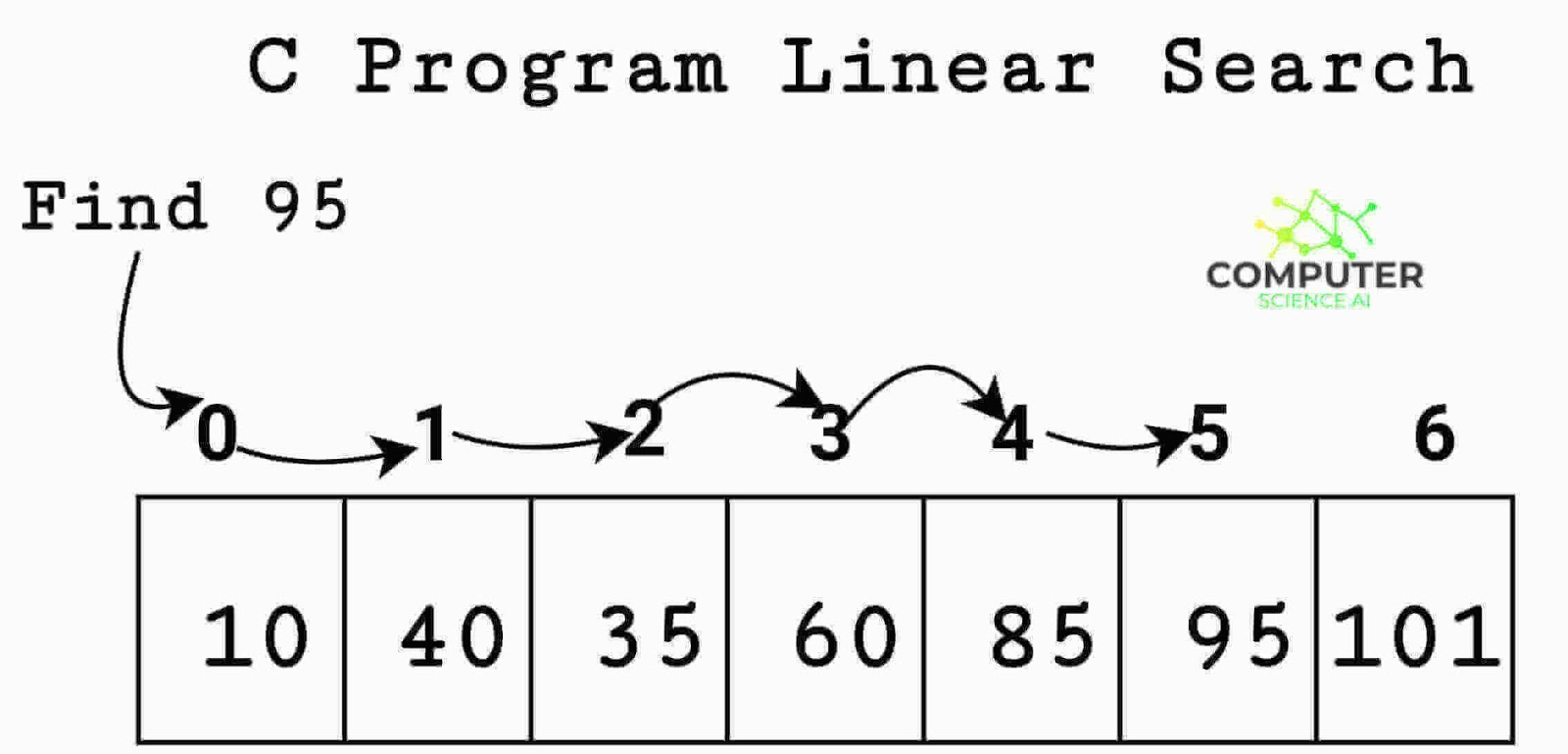# C Program for Linear Search

C program for linear search: This c program uses linear search algorithm to find the location or index of an element in given array.#### Linear Search C Programs

``````#include<stdio.h>

// Linearly search x in the array[] and
// if x is present then return its position
// otherwise return -1

int linear_search(int arr[], int size, int x)
{
int i;

for (i = 0; i < size; i++)
if (arr[i] == x)
return i;

return -1;
}

int main()
{

int arr[] = {10,40,35,60,85,95,101};

int n = sizeof(arr) / sizeof(arr);

int x = 95;

int location_index = linear_search(arr, n, x);

if(location_index == -1)
printf("Element is not present in the given array");
else
printf("Element is present at %d th location or index in given array",location_index+1);
return 0;
}

/* Output of above code:-

Element is present at 6th location or index in the givem array

*/``````

## Linear search in C using function

This code implements a linear searching algorithm to find whether a city name is present in a file or not and if it is present at what location it occurs. Linear search is also known as a sequential search. Working of this algorithm is very simple: We compare each element of the array or file with the element to be found from the beginning until it is found.

Here is the source code of C program for linear search using function
```/* Aim: C program for linear search of string */

#include<stdio.h>
#include<stdlib.h>
#include<string.h>

int linearSearch(char city[],int size,char key)
{
int i;

for(i=0;i<size;i++)
if(strcmp(*(city + i),key)==0);
return i+1;

return -1;
}

int main()
{
FILE *fp;
int size,i=0,pos;
char cityName,key,c=' ';

fp=fopen("city.txt","r");

if(fp==NULL)
exit(0);
else
{
while(!feof(fp))
{
fscanf(fp,"%s",cityName[i]);
i++;
}
}

size=i-1;

printf("\n Enter the name of city to search for:- ");
scanf("%s",key);

pos=linearSearch(cityName,size,key);

if(pos==-1)
printf("\n There is no city named %s in city.txt \n",key);
else
printf("\n %s is present in city.txt at %d no position. \n \n",key,pos);

return 0;
}

/* Output of above code / Runtime test cases:-

Enter the name of city to search for:- Queens

Queens is present in city.txt at 5 no position.

*/

```

C program for binary search

## C program for Linear search for multiple occurrences

This code prints all locations in an array where the element to be searched is present, finally it prints the element with total count.
``````
#include<stdio.h>

int main()
{
int search_element, array, i, n, count = 0;

printf("\n How many elements to accept in the array:- ");
scanf(" %d", &n);

printf("\n Enter %d numbers:- ", n);

for(i=0;i<n;i++)
scanf(" %d", &array[i]);

printf("\n Enter any number to search in array:- ");
scanf(" %d", &search_element);

for(i=0;i<n;i++)
{
if(array[i]==search_element)
{
printf("\n %d is present at location %d ", search_element, i+1);
count++;
}
}

if(count==0)
printf("\n %d is not present in the array. \n", search_element);
else
printf("\n %d is present at %d times in the array \n", search_element, count);

return 0;
}

/* Output of above code:-

How many elements to accept in the array:- 5

Enter 5 numbers:- 45 90 78 40 45

Enter any number to search in array:- 45

45 is present at location 1
45 is present at location 2

45 is present 2 times in the array

*/``````

## Function for linear search using pointers

```long Linear_Search(long size, long element, long *pointer)
{
long i;

for(i=0;i<size;i++)
{
if(*(pointer+i)==element)
return i;
}

return -1;
}```